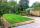# Rectangle - sides 3

If in the rectangle ABCD we enlarge the side a by 5 cm and decrease the side b by 2 cm, the rectangle area will be reduced by 5 cm². When we decrease the length of the side a by 4 cm and and at the same time we increase the length of side b by 3 cm we increases the area of the original rectangle by 5 cm².

Calculate the length of the sides of the rectangle ABCD.

Result

a =  15 cm
b =  7 cm

#### Solution:

(a+5)*(b-2) = a*b - 5
(a-4)*(b+3) = a*b + 5

2a-5b = -5
3a-4b = 17

a = 15
b = 7

Calculated by our linear equations calculator.

Leave us a comment of example and its solution (i.e. if it is still somewhat unclear...):Be the first to comment!#### To solve this example are needed these knowledge from mathematics:

Do you have a system of equations and looking for calculator system of linear equations?

## Next similar examples:

1. Rectangle vs squareOne side of the rectangle is 1 cm shorter than the side of the square, the second side is 3 cm longer than the side of the square. Square and rectangle have the same content. Calculate the length of the sides of a square and a rectangle.
2. Rectangles - sidesOne side of the rectangle is 10 cm longer than second. Shortens longer side by 6 cm and extend shorter by 14 cm increases the area of the rectangle by 130 cm2. What are the dimensions of the original rectangle?
3. Rectangle - sides ratioCalculate area of rectangle whose sides are in ratio 3:13 and perimeter is 673.
4. Rectangular fieldOne dimension of the rectangular field is 56 m greater than second dimension. If each side of the rectangle increases by 10 m, increases the surface field is 1480 m2 . Determine dimencions of the field.
5. PropertyThe length of the rectangle-shaped property is 8 meters less than three times of the width. If we increase the width 5% of a length and lendth reduce by 14% of the width it will increase the property perimeter by 13 meters. How much will the property cost
6. The hallThe hall had a rectangular ground plan one dimension 20 m longer than the other. After rebuilding the length of the hall declined by 5 m and the width has increased by 10 m. Floor area increased by 300 m2. What were the original dimensions of the hall?
7. Three shapes1/5 of a circle is shaded. The ratio of area if square to the sum of area of rectangle and that of the circle is 1:2. 60% of the square is shaded and 1/3 of the rectangle is shaded. What is the ratio of the area of circle to that of the rectangle?
8. GardenThe garden has a rectangular shape and has a circumference of 130 m and area 800.25 m2. Calculate the dimensions of the garden.
9. Two squaresTwo squares whose sides are in the ratio 5:2 have sum of its perimeters 73 cm. Calculate the sum of area this two squares.
10. Triangle ABCCalculate the sides of triangle ABC with area 1404 cm2 and if a: b: c = 12:7:18
11. Trapezoidtrapezoid ABCD a = 35 m, b=28 m c = 11 m and d = 14 m. How to calculate its area?
12. Diamond diagonalsCalculate the diamond's diagonal lengths if its content is 156 cm2 and the side length is 13 cm.
13. Right triangle AlefThe obvod of a right triangle is 84 cm, the hypotenuse is 37 cm long. Determine the lengths of the legs.
14. Circle arcCircle segment has a circumference of 135.26 dm and 2096.58 dm2 area. Calculate the radius of the circle and size of central angle.
15. Isosceles triangleThe perimeter of an isosceles triangle is 112 cm. The length of the arm to the length of the base is at ratio 5:6. Find the triangle area.
16. Isosceles trapezoidCalculate the content of an isosceles trapezoid whose bases are at ratio 5:3, the arm is 6cm long and it is 4cm high.
17. TrapezoidHow long are the trapezoid bases with area 24 cm2 and height 3 cm. One base is 3 times longer than the shorter.